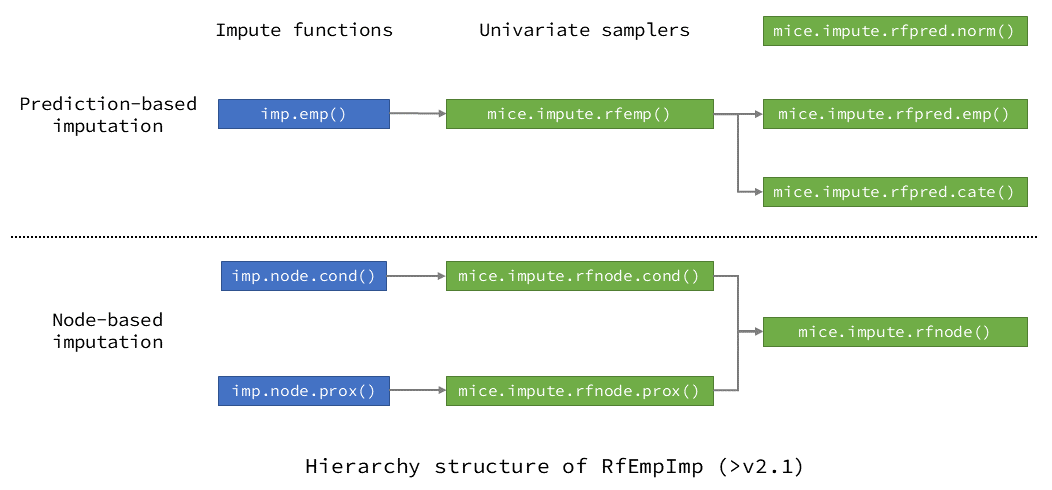# Introduction to RfEmpImp

An R package for random-forest-empowered imputation of missing Data

## Random-forest-based multiple imputation evolved

RfEmpImp is an R package for multiple imputation using chained random forests (RF).
This R package provides prediction-based and node-based multiple imputation algorithms using random forests, and currently operates under the multiple imputation computation framework mice.
For more details of the implemented imputation algorithms, please refer to: arXiv:2004.14823 (further updates soon).

## Installation

Users can install the CRAN version of RfEmpImp from CRAN, or the latest development version of RfEmpImp from GitHub:

# Install from CRAN
install.packages("RfEmpImp")
# Install from GitHub online
if(!"remotes" %in% installed.packages()) install.packages("remotes")
remotes::install_github("shangzhi-hong/RfEmpImp")
# Install from released source package
install.packages(path_to_source_file, repos = NULL, type = "source")
# Attach
library(RfEmpImp)

## Prediction-based imputation

### For mixed types of variables

For data with mixed types of variables, users can call function imp.rfemp() to use RfEmp method, for using RfPred.Emp method for continuous variables, and using RfPred.Cate method for categorical variables (of type logical or factor, etc.).
Starting with version 2.0.0, the names of parameters were further simplified, please refer to the documentation for details.

### Prediction-based imputation for continuous variables

For continuous variables, in RfPred.Emp method, the empirical distribution of random forest’s out-of-bag prediction errors is used when constructing the conditional distributions of the variable under imputation, providing conditional distributions with better quality. Users can set method = "rfpred.emp" in function call to mice to use it.

Also, in RfPred.Norm method, normality was assumed for RF prediction errors, as proposed by Shah et al., and users can set method = "rfpred.norm" in function call to mice to use it.

### Prediction-based imputation for categorical variables

For categorical variables, in RfPred.Cate method, the probability machine theory is used, and the predictions of missing categories are based on the predicted probabilities for each missing observation. Users can set method = "rfpred.cate" in function call to mice to use it.

### Example for prediction-based imputation

# Prepare data
df <- conv.factor(nhanes, c("age", "hyp"))
# Do imputation
imp <- imp.rfemp(df)
# Do analyses
regObj <- with(imp, lm(chl ~ bmi + hyp))
# Pool analyzed results
poolObj <- pool(regObj)
# Extract estimates
res <- reg.ests(poolObj)

## Node-based imputation

For continuous or categorical variables, the observations under the predicting nodes of random forest are used as candidates for imputation.
Two methods are now available for the RfNode algorithm series.
It should be noted that categorical variables should be of types of logical or factor, etc.

### Node-based imputation using predicting nodes

Users can call function imp.rfnode.cond() to use RfNode.Cond method, performing imputation using the conditional distribution formed by the prediction nodes.
The weight changes of observations caused by the bootstrapping of random forest are considered, and only the “in-bag” observations are used as candidates for imputation.
Also, users can set method = "rfnode.cond" in function call to mice to use it.

### Node-based imputation using proximities

Users can call function imp.rfnode.prox() to use RfNode.Prox method, performing imputation using the proximity matrices of random forests.
All the observations fall under the same predicting nodes are used as candidates for imputation, including the out-of-bag ones.
Also, users can set method = "rfnode.prox" in function call to mice to use it.

### Example for node-based imputation

# Prepare data
df <- conv.factor(nhanes, c("age", "hyp"))
# Do imputation
imp <- imp.rfnode.cond(df)
# Or: imp <- imp.rfnode.prox(df)
# Do analyses
regObj <- with(imp, lm(chl ~ bmi + hyp))
# Pool analyzed results
poolObj <- pool(regObj)
# Extract estimates
res <- reg.ests(poolObj)

## Imputation functions

Type Impute function Univariate sampler Variable type
Prediction-based imputation imp.emp() mice.impute.rfemp() Mixed
/ mice.impute.rfpred.emp() Continuous
/ mice.impute.rfpred.norm() Continuous
/ mice.impute.rfpred.cate() Categorical
Node-based imputation imp.node.cond() mice.impute.rfnode.cond() Mixed
imp.node.prox() mice.impute.rfnode.prox() Mixed
/ mice.impute.rfnode() Mixed

## Package structure

The figure below shows how the imputation functions are organized in this R package.## Support for parallel computation

As random forest can be compute-intensive itself, and during multiple imputation process, random forest models will be built for the variables containing missing data for a certain number of iterations (usually 5 to 10 times) repeatedly (usually 5 to 20 times, for the number of imputations performed). Thus, computational efficiency is of crucial importance for multiple imputation using chained random forests, especially for large data sets.
So in RfEmpImp, the random forest model building process is accelerated using parallel computation powered by ranger. The ranger R package provides support for parallel computation using native C++. In our simulations, parallel computation can provide impressive performance boost for imputation process (about 4x faster on a quad-core laptop).